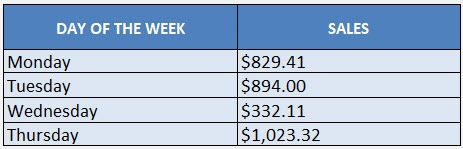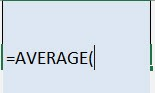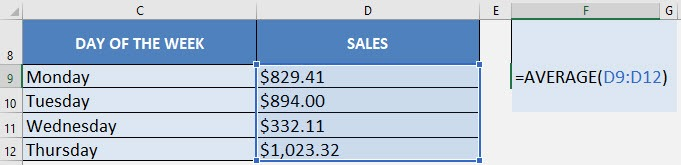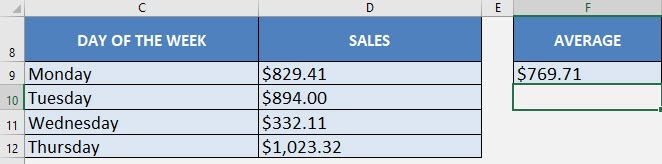What does it do?

Gives you the average of a group of values

Formula breakdown:

=AVERAGE(number1, number2…)

What it means:

=AVERAGE(the numbers you want to average)

There are times when you have to get the average of your values in your Excel worksheet and you would normally have to SUM all of the values then divide it by the number of values.

That’s the long process!

Thankfully there is a quicker way with Excel’s AVERAGE formula!

In our example below, we have the table of values that we need to get the average from:I explain how you can do this below:STEP 1: We need to enter the AVERAGE  function in a blank cell:

## =AVERAGE(STEP 2: The AVERAGE arguments:

## text

What numbers do we want to get the average of?

Select the range of values:

## =AVERAGE(D9:D12)You have now calculated the average of the Sales numbers!Get the Average of Values with Excel’s AVERAGE Formula